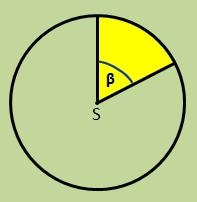# Calculate 8325

Calculate the area of a circular section given by an angle of 220 degrees if the circle's radius is 20cm. Round the result to cm2

S =  768 cm2

### Step-by-step explanation:Did you find an error or inaccuracy? Feel free to write us. Thank you!

Tips for related online calculators
Do you want to round the number?

#### Grade of the word problem:

We encourage you to watch this tutorial video on this math problem: